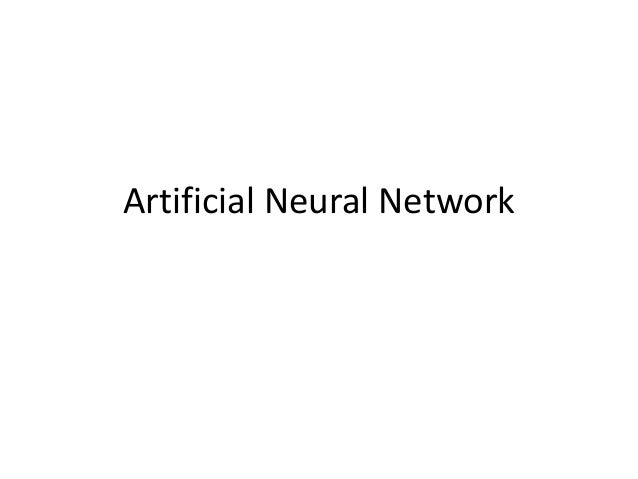Successfully reported this slideshow.
We use your LinkedIn profile and activity data to personalize ads and to show you more relevant ads. You can change your ad preferences anytime.Upcoming SlideShare
Loading in …5
×

# A brief introduction of Artificial neural network by example

A simple introduction with a solved example about artificial neural networks.Beginners can use this tutorial to gain a basic understanding about the ANN architecture and the process by which ANN model is developed for practical problem solving.The example in the tutorial describe the way ANN models are developed.ANN is widely popular and used in various artificial intelligence and internet of things projects.

• Full Name
Comment goes here.

Are you sure you want to Yes No
Your message goes here• Be the first to comment

### A brief introduction of Artificial neural network by example

1. 1. Artificial Neural Network
2. 2. Definition • Artificial Neural network or ANN is a very popular method for predictive or optimization or simulation objectives. • ANN mimics the human nervous system to solve problems in a parallel manner. • ANN are known to be adaptable with situations, flexible with data and efficient enough for predicting any kind of problems.
3. 3. Mathematical Representation of ANN Single Layer Neural Network Multi Layer Neural Network
4. 4. Mathematical Representation of ANN Single Layer Neural Network Multi Layer Neural Network Yj = gA ( ∑hij x Xi + aj ) Where Y ,Z are the output hj are the hidden nodes and Xi are the inputs, f and g are the activation function for the hidden to output and input to hidden layers respectively, Xi are the inputs, w and h are the weights of respectively for hidden to output and input to hidden layer connections and a,b are the bias. i = 1…n,j = 1…h. Zk = fA ( ∑wjk x hj + bk) and hj = gA (∑ hij x Xi + aj ) Eqn 1 Eqn 2 Eqn A
5. 5. Basic Methodology of ANN 1. Selection of the Model Topology : ANN have an input and output layer. Between this two layers, lies the hidden layer which actually separates the ANN model from the other linear and non-linear models. Selection of the number of hidden layers influences the efficiency of the model. More the number of hidden layers more complex but efficient will be the model and vice versa. 2. Training for determination of the optimal value of the weights. The weight of the inputs are changed to equate the predicted value with the desired value of the output. Whenever both the desired and predicted value becomes equal or nearly equal to the satisfaction of the developer the training is stopped. 3. Validation of the Model by predicting the known outputs.
6. 6. Problem 1 • A two input-one output model is required to be developed. The training data for the model is as follows : • Input 1 : 10 • Input 2 : 12 • Output 1 : 24. • Find the value of the output when Input 1 is 5 and Input 2 is 7 if number of hidden layer is 1 and node is 2.
7. 7. Solution • The architecture of the ANN will be as below : Input 1 Input 2 Hidden 1 Hidden 2 Output 1 h 11 h 12 h 21 h 22 w 11 w 21
8. 8. Solution • As the ANN will be multilayer : Input, Hidden and Output layers we will use Eqn 1 and Eqn 2 to find the optimal weights first. • Then we will use the optimal weights in the same equation to find the output or answer of the problem
9. 9. Solution • Zk = fA ( ∑wjk x hj + bk) And • hj = gA (∑ hij x Xi + aj ) Here X1 and X2 is 10 and 12 respectively And Z1 is 24 Let hij the weights be 0.5 and wjk be 05.
10. 10. Solution • Replacing we have : • Eqn 2 = • h1 = ga(h11X10+h21X12)+ a1) • if ga = Logarithmic function(aLog(X)) and a1 is negligible then taking h11 and h22 as 0.5 will give the following : • 10xLOG (0.5x10+0.5x12) = 10xLOG(5+6) = 10xLOG(11) =10.41 = h1 • Similarly h2 will also be 10.41
11. 11. Solution • As, Zk = fA ( ∑wjk x hj + bk) • Then we can write : • Eqn 1 = • Z1 = fA ( 0.5xh1 + 0.5xh2) + b1 ) • If fA is taken as Logarithmic and we know that h1 = h2 = 10.41 • then • Z1 = 10xLog(0.5x10.41+0.5x10.41) • = 10.17 = Eqn.1
12. 12. Solution • Now the desired or given output is 24 • So Absolute Error or AE = 24-10.17 =13.82 • So we have to change the value of h and w and recalculate the output. • When the AE is less than 20% the value of weights (h and w) will be taken as optimal. • The same Eqn 1 and 2 will be used. This time we know the value of inputs and weights but do not know the value of output.
13. 13. Solution • Eqn 2 = • h1 = ga(h11X5+h21X7)+ a1) • if ga = Logarithmic function and a1 is negligible and optimal value h11 and h22 as 1.5 will give the following : • 10xLOG (1.5x5+1.5x7) = 12.55 = h1 • Similarly h2 will also be 12.55
14. 14. Solution • As, Zk = fA ( ∑wjk x hj + bk) • Then we can write : • Eqn 1 = • Z1 = fA ( 1.5xh1 + 1.5xh2) + b1 ) • If fA is taken as Logarithmic and we know that h1 = h2 = 12.55 • then • Z1 = 10xLog(1.5x12.55+1.5x12.55) • = 15.75 = Eqn.1 • So the output or answer is 15.75.